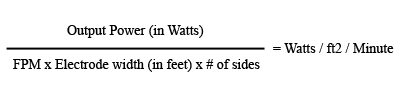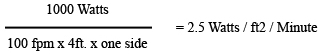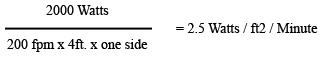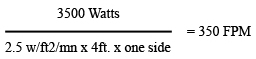Create Config
Edit Name Description Configiration Type Type

# Watt Density

## What Is The Formula To Calculate Watt Density?

Watt Density is a measurement of the amount of energy being applied to the web. It is measured in Watts/Foot2/minute. Watt density takes into account the amount of power being applied (watts), the time it is being applied (minute) and the amount of material it is being applied to (Foot2).

Once the watt density is known to get a particular material to a certain dyne level, it can be used to predict the results if any of the parameters change such as line speed.

### The formula to calculate watt density is as follows:Example: A line is running at 100 feet per minutes (fpm), the power supply output is 1kW and 45 dyne is being achieved. you can expect the exact same results at 200 fpm at 2kW 300 fpm at 3kW, etc.

If the example above were a 48 inch treat width, one side treat – a watt density of 2.5 watts/ Ft2/minute is required obtain 45 dyne.(Electrode width = 4feet)OR## The Same Formula Can Be Used To Determine:

### 1. Required Power at a certain line speed.

Using the same example, lets say you would like to increase your line speed to 500 FPM and still get 45 dyne on the same material, the formula can be used as follows:

500 fpm x 4ft x one side x 2.5 Watts/Ft2/Min. = 5000 watts

5Kw is required to get 45 dyne at 500fpm.

### 2. Maximum speed capacity:

Using the same example lets say you have a 3.5Kw power supply and you would like to know the fastest line speed you can achieve and still get 45 dyne. The formula can be used as follows: